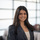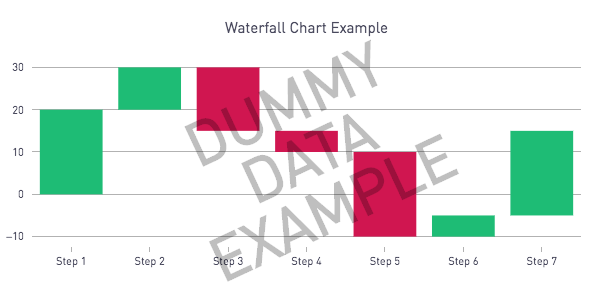# Waterfall Charts — Plot.lyWaterfall charts are a great way to highlight changes in your data between steps or periods. The following python script easily plugs in with your SQL output, simply paste this into your Python 3.6 code box provided with the Python/R Integration, ensuring that your SQL output has 2 columns:

1. x_data: the values along the x axis
2. y_data: the y value for each x. Note that this is not the change between x and y, but rather the final value of y at each step

Code is inspired from the examples on plot.ly's documentation. Refer to the documentation for further customization options,

Python 3.6 Code

```# PERISCOPE WATERFALL TEMPLATE
# SQL output should have 2 columns:
#    1) x_data: the values along the x axis
#    2) y_data: the y value for each x. Note that this is not the change between x and y, but rather the final value of y at each step

# Import libraries
import pandas as pd
import plotly.plotly as py
import plotly.graph_objs as go

# Create Dummy data
x_data = ['Step 1', 'Step 2','Step 3', 'Step 4','Step 5', 'Step 6', 'Step 7']
y_data = [20, 30, 15, 10, -10, -5, 15]
data = {'x_data':x_data, 'y_data':y_data}
dummy_df = pd.DataFrame(data)
community_post = 'https://community.periscopedata.com/t/630sck'

# HELPER FUNCTION: For annotation text
style = ';'.join([f'{key.replace("_","-")}:{settings[key]}' for key in settings])
return f'<a href="{link}" style="{style}">{text}</a>'

# MAIN FUNCTION: input a dataframe
def plot(df, annotation = None):

# Split our dataframe for easier work
x_data = df['x_data']
y_data = df['y_data']

# Initiate y_bottom array to denote the starting point for all the waterfall bars.
y_bottom = 

# Initiate the y_length array to enote the length of the waterfall "drop"
y_length = [y_data]

# Initiate a color array that will show red bars for negative change and green bars for positive change
if (y_data > 0):
y_color = ['rgba(33,196,128,1.0)']
else:
y_color = ['rgba(214,24,90,1.0)']

# Calculate remaining bar positioning and appropriate colors, green for a positive change and red for a negative change
for i in range(1,len(y_data)):
dist = y_data[i] - y_data[i-1]
length = abs(dist)
y_length.append(length)
if (y_data[i] > y_data[i-1]):
bottom = y_data[i-1]
color = 'rgba(33,196,128,1.0)'
else:
bottom = y_data[i-1] - length
color = 'rgba(214,24,90,1.0)'
y_bottom.append(bottom)
y_color.append(color)

# CREATE PLOT.LY GRAPH

# bottom_of_bar is a transparent series. The length bar stacks on top of the bottom_of_bar
bottom_of_bar = go.Bar(
x = x_data,
y = y_bottom,
marker=dict(
color='rgba(0,0,0, 0.0)',
)
)
# length_of_bar stacks on top of the bottom_of_bar
length_of_bar = go.Bar(
x=x_data,
y = y_length,
marker=dict(
color = y_color
)
)

# Putting our data together
data = [bottom_of_bar, length_of_bar]

# Formatting includes chart titles and margin sizing
layout = go.Layout(
title='Waterfall Chart Example',
barmode='stack',
showlegend=False,
margin=dict(
l=50,
r=50,
b=50,
t=50
)
)

# Add an annotation if the SQL output is in the incorrect format
if annotation is not None:
layout['annotations'] = [annotation]

# Plot the figure
fig = go.Figure(data=data, layout=layout)
periscope.plotly(fig)

# We try to to plot the SQL output. If it is not in the correct format, the dummy data will display with a watermark. See stdout tab for the error message.
try:
plot(df)
except Exception as e:
print(e)
annotation = {
'x': 0.5,
'y': 0.5,
'ax': 0,
'ay': 0,
'xref': 'paper',
'yref': 'paper',
'text': style_link('DUMMY<br><br><br><br>DATA<br><br><br><br>EXAMPLE', community_post, font_size='60px', font_weight='bold', color='rgba(0, 0, 0, .25)'),
'showarrow': False,
'textangle': -25
}
plot(dummy_df, annotation=annotation)
```

Don't have access to plot.ly? You can create something similar using Periscope's default charting libraries!